07变量，自定义姓名，好感度，flag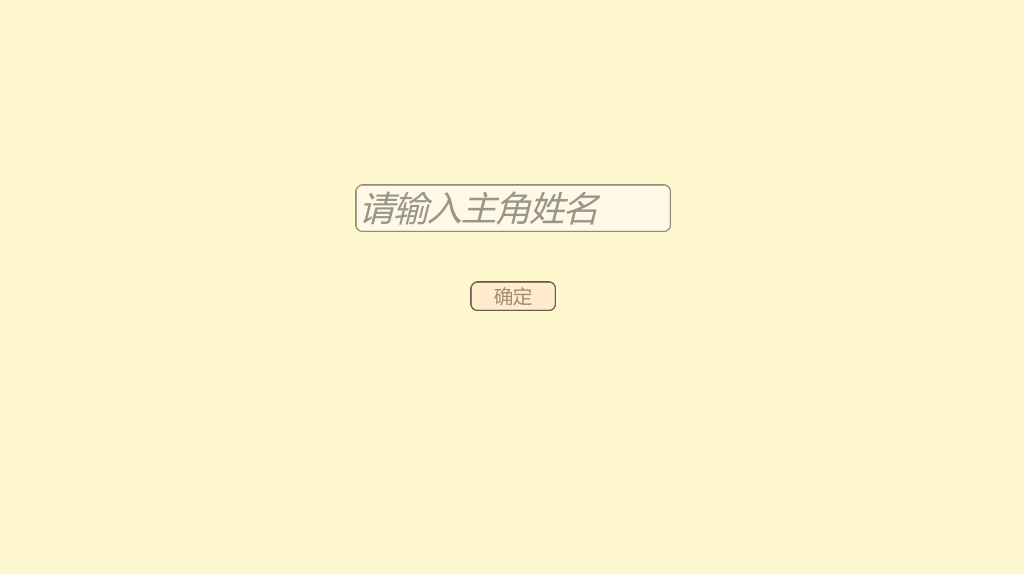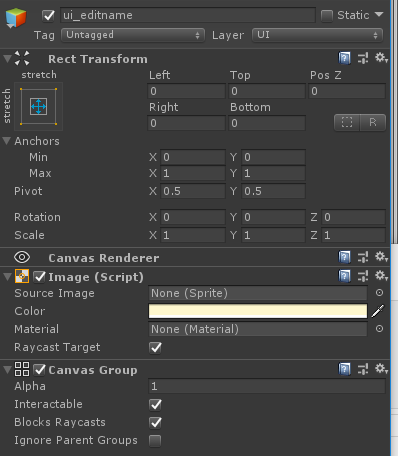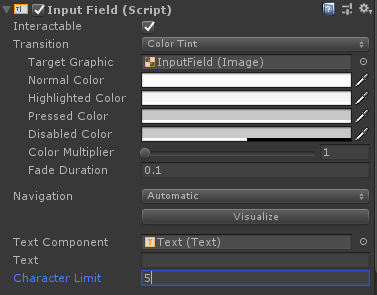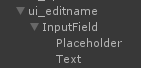Placeholder是用来显示输入提示文字的，我们将它的文字改为“请输入主角姓名”，并且将文字的字号和对齐等适当的改成自己想要的样子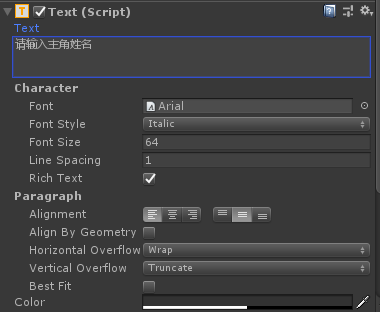{

GameObject temp = GameObject.Find("ui_editname");

Animator an = temp.GetComponent<Animator>();

an.SetInteger("a_num", 1);

}

{

GameObject temp = GameObject.Find("ui_editname");

Animator an = temp.GetComponent<Animator>();

an.SetInteger("a_num", 2);

StartCoroutine(waittime(1f));

}

@selectfadein text1=吃 text2=不吃 target1=*t1 target2=*t2

*t1

@jumpscript target=*c1

*t2

*c1

//建立一个用来储存游戏变量的字典，为了让用krkr的人感觉更亲切一些，我给这个字典起名叫f
Dictionary<string, string> f = new Dictionary<string, string>();
{

GameObject temp = GameObject.Find("ui_editname");

//将玩家输入的姓名起名叫name放入储存变量的字典里，字典里的变量名字（key）也可以是中文的，不过还是推荐使用英文。
f["name"] = temp.transform.Find("InputField").gameObject.GetComponent<InputField>().text;

Animator an = temp.GetComponent<Animator>();
an.SetInteger("a_num", 2);
StartCoroutine(waittime(1f));

}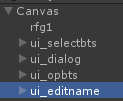canvas下边放了这么多东西就是会这样的啊哈哈哈哈，虽然所有的界面默认状态都是透明的，但是他们依然会拦截点击事件，ui_opbt在ui_editname下边，所以就被ui_editname挡住了，虽然ui_editname现在是透明，但是就像是一块透明玻璃板一样挡住了鼠标的点击，会导致下边的界面全部都无法点击的。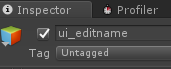{

//以往用的Gameobject.Find无法获取active是false的gameobject，一定要先获取ui_editname的父object “Canvas”，然后通过它的transform来寻找到没有被激活的ui_editname

GameObject temp = GameObject.Find("Canvas").transform.Find("ui_editname").gameObject;

//将它激活

temp.SetActive(true);

Animator an = temp.GetComponent<Animator>();

an.SetInteger("a_num", 1);

}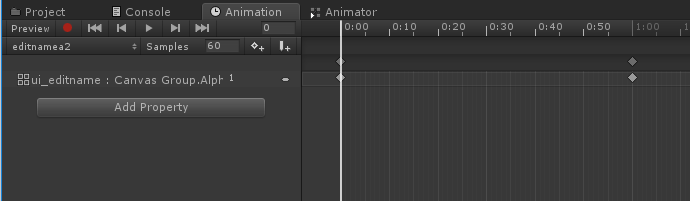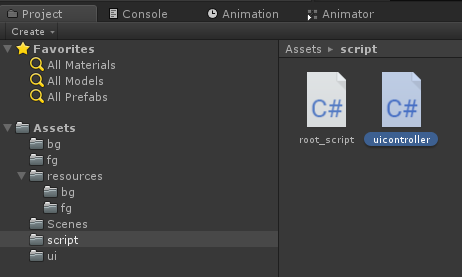using System.Collections;

using System.Collections.Generic;

using UnityEngine;

public class uicontroller : MonoBehaviour {

// Use this for initialization

void Start () {

}

// Update is called once per frame

void Update () {

}

public void activefalse()

{

//这里的this指的是挂载了这个c#脚本的gameobject，比如把它挂在ui_editname上，这个this就指的是ui_editname，挂在ui_dialog上指的就是ui_dialog，一个脚本可以挂在多个gameobject上，他们的行为也不会相互影响

this.gameObject.SetActive(false);

}

}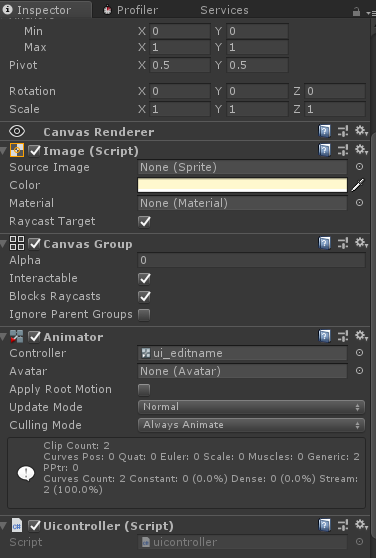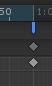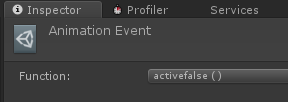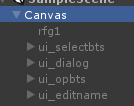//建立一个用来储存游戏变量的字典，为了让用krkr的人感觉更亲切一些，我给这个字典起名叫f
Dictionary<string, string> f = new Dictionary<string, string>();

{

GameObject temp = GameObject.Find("ui_editname");

//将玩家输入的姓名起名叫name放入储存变量的字典里，字典里的变量名字（key）也可以是中文的，不过还是推荐使用英文。

f["name"] = temp.transform.Find("InputField").gameObject.GetComponent<InputField>().text;

//如果传入的名字是空，就不执行下边的淡出动画

if (f["name"] =="")

{

return;

}

Animator an = temp.GetComponent<Animator>();

an.SetInteger("a_num", 2);

StartCoroutine(waittime(1f));

}

void ch(Dictionary<string ,string>elm)

{

dtext = dtext + f[elm["text"]];

startscript();

}

@ch text=name

，你要吃包子吗？

@ch text=atk

dtext =  line;

dtext = dtext + line;

{

GameObject temp = GameObject.Find("ui_dialog_text");

//将dtext里边存的文字赋值给画面,为了能够使用Text，需要在文件头加上using UnityEngine.UI;

temp.GetComponent<Text>().text = dtext;

dtext = "";

Animator an = temp.GetComponent<Animator>();

an.SetInteger("a_num", 1);

//设定开始等待点击

onwaitclick = true;

}

@ch text=name

，你要吃包子吗？

@selectfadein text1=吃 text2=不吃 target1=*t1 target2=*t2

*t1

@jumpscript target=*c1

*t2

*c1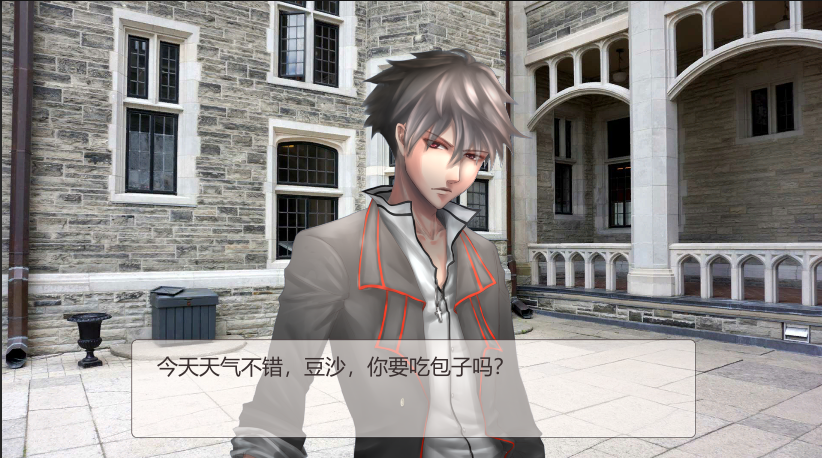void initf()

{

//c#是强类型语言，因为我们的字典在定义的时候是<string,string>也就是名字和值都是字符串，所以即使是好感度，也是要用”0”这个字符串而不是0来保存。在使用的时候也要再临时将字符串转换成数值才能使用。

f["xyfeel"] = "0";

startscript();

}

@initf

@ch text=name

，你要吃包子吗？

@selectfadein text1=吃 text2=不吃 target1=*t1 target2=*t2

*t1

@jumpscript target=*c1

*t2

*c1

using System;

{

double temp = Convert.ToDouble(f[elm["tar"]]);

temp = temp + add;

f[elm["tar"]] = temp.ToString();

startscript();

}

@initf

@ch text=name

，你要吃包子吗？

@selectfadein text1=吃 text2=不吃 target1=*t1 target2=*t2

*t1

@jumpscript target=*c1

*t2

*c1

@ch text=xyfeel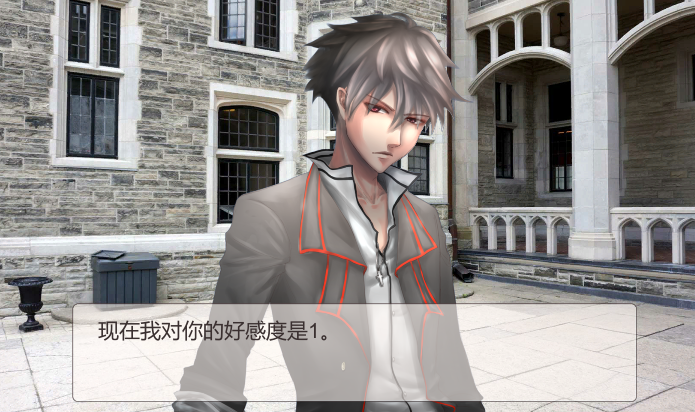void initf()

{

f["xyfeel"] = "0";

f["xyflag1"] = "0";

startscript();

}

@initf

@ch text=name

，你要吃包子吗？

@selectfadein text1=吃 text2=不吃 target1=*t1 target2=*t2

*t1

@jumpscript target=*c1

*t2

*c1

@selectfadein text1=梅菜肉包 text2=白菜肉包 target1=*t3 target2=*t4

*t3

@jumpscript target=*c2

*t4

*c2

@selectfadein text1=喜欢 text2=不喜欢 target1=*t5 target2=*t6

*t5

@jumpscript target=*c3

*t6

*c3

@checkend

*end1

@jumpscript target=*c4

*end2

*c4

void checkend()

{

double feel = Convert.ToDouble(f["xyfeel"]);

double flag = Convert.ToDouble(f["xyflag1"]);

//只有好感度有2以上且触发了flag1的情况下才会进入end1，其他情况进入end2

if(feel>=2&&flag==1)

{

jumpscript1("*end1");

}

else

{

jumpscript1("*end2");

}

}

aa